×

Search anything:

# md5sum and sha256sum commands in Linux

#### Linux Software EngineeringGet this book -> Problems on Array: For Interviews and Competitive Programming

MD5 (message-digest algorithm) and SHA-256 are hashing algorithms that take in a message and produce a fixed-length digest/hash we can use to verify the integrity of a file or directory. We learn about md5sum and sha256sum commands in Linux.

1. Introduction.
2. Syntax.
3. Commands usage.
4. Summary.
5. References.

## Introduction.

MD5(message-digest algorithm) and SHA-256 are hashing algorithms that take in a message and produce a fixed-length digest we can use to verify the integrity of a file or directory.

In Linux, we use the md5sum command which uses the MD5 algorithm to validate a checksum. The command puts the specified file through the MD5 algorithm to produce a checksum. This is the same for sha256sum command except that it uses the SHA-256 algorithm which is more secure and has fewer collisions compared to the former

There are many use cases where we would use these commands. Some are listed below:

• To verify the validity of a downloaded file, in this case, we compare the checksum of the original version with the checksum of the downloaded version. If they match then the file is correct otherwise it is corrupt.
• We can also use this command to verify the checksums of two versions of a backup. While we make incremental backups to files and directories, we may want to be sure that both versions match. For this, we compare the checksums of both backups. If they match it means that the data was replicated successfully.
• We use the scp command to transfer files securely between remote systems, sometime, the file or program might be working in the source location but presents issues in the destination location. In such cases, the issues usually lie in the transmission of the file. Here we compare the two to verify the integrity of the files.
• Sometimes we have large files we need to transfer between two remote hosts and although compression is an option, at times, the compressed file might still be very taxing to bandwidth. In such cases, we can divide the compressed file and send it in chunks then reassemble the chunks to form the complete original file. Once this operation is done, we should compare the checksums of the two files to make sure no data was lost in the process.

## Syntax.

We write md5sum commands using the following syntax.

``````\$ md5sum [OPTION]... [FILE]...
``````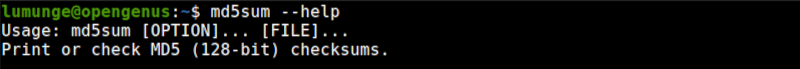We write sha256sum commands using the following syntax:

``````\$ sha256sum [OPTION]... [FILE]...
``````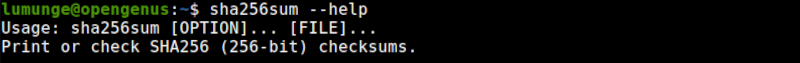## Command usage.

1. To generate the checksum of a file we execute the command:
Using the MD5 algorithm:
``````\$ md5sum [file]
``````

In the above example, we get the checksum of a snapshot of the home directory.

Using SHA-256 algorithm:

``````\$ sha256 [file]
``````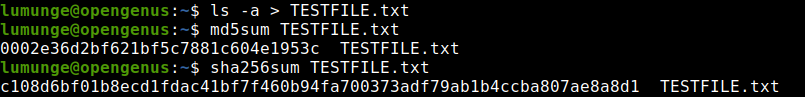In the above screenshot, we use both md5sum and sha256sum commands to generate a message digest or hash for the file TESTFILE.txt which has the output from the ls -a command.

We can change the contents of the file and generate another checksum.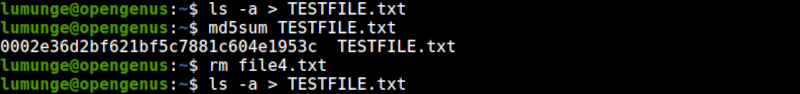Above we generate the checksum for the original file then remove a file - file4.txt that has no content. This action will change the contents of TESTFILE.txt. After this operation, we redirect the ls -a command output to TESTFILE.txt.

Next, we generate another checksum for the same file. Notice that the checksum differs from the original.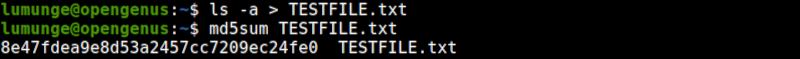Now, let's recreate the file we previously deleted - file4.txt using the touch command, and use ls -a command which redirects its output to TESTFILE.txt. When we generate the checksum for the file again, we find that it is similar to the original file.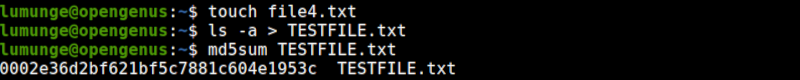This goes to show that when using MD5 algorithm, two files with different contents rarely have the same checksum.

Also, note that changing the name of the file won't affect the checksum value as it is derived from the file contents and not the file name

Here is the whole process: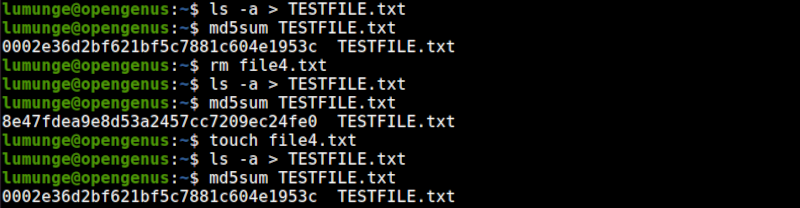We can also try this with SHA-256 algorithm. We should expect the same behavior.

1. The default mode to view the checksum of a file is in text mode. We can also view it in binary by using the -b option:
``````\$ md5sum -b [file]
``````

We can also create a BSD style checksum using the --tag option as follows:

``````\$ md5sum --tag [file]
``````

And using SHA-256 we write:

``````\$ sha256sum --tag [file]
``````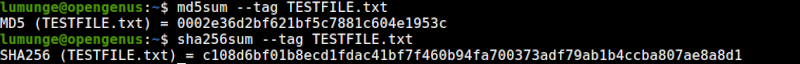1. Now to compare checksums between two files.
``````\$ md5sum -c [copied file]
``````

Here we assume we have copied files or downloaded a file from the internet. Some downloads provide a checksum file which we can use to verify the integrity of a file before executing it in our system.

We use the -c option to check the checksums and compare them. If they match, then we expect an OK message to be printed out, otherwise, the command will report a failed checksum matching.

We can also ssh to a remote server and execute this command there.

An example is shown below: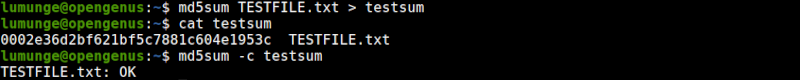First, we have to generate the checksum of the original file and store it in another file - testsum. We do this using I/O redirection.
After the above operation, our checksum for TESTFILE.txt is stored in the file testsum. To compare the checksum we write:

``````\$ md5sum -c testsum
``````

An example of a failed case is shown below: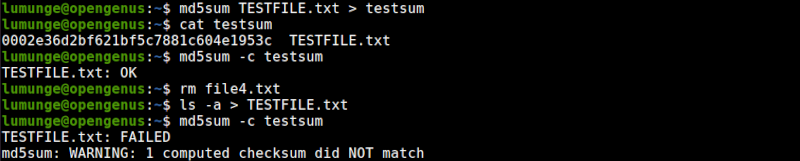Here, we remove a file - file4.txt and generate another TESTFILE.txt using the ls -a command. This file is however different from the original since we deleted a file.
As we can see we have a warning informing us the checksum did not match, meaning the current file is corrupted.

1. We can also perform the above action across multiple files by saving the files checksums inside a common file and passing the file to the md5sum command.
``````\$ md5sum -c commonfile
``````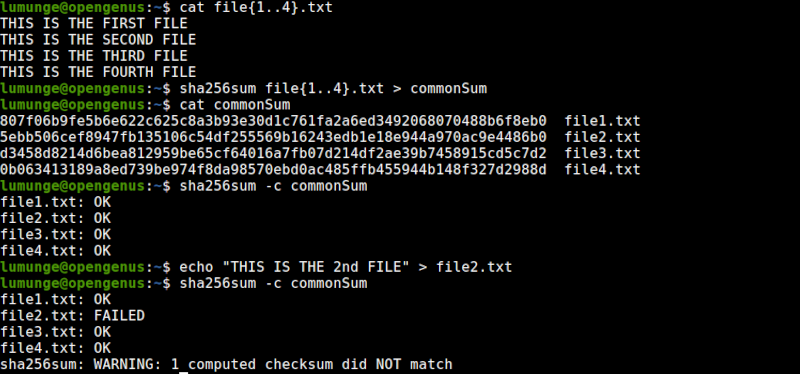In this example, we generate the checksums of four files using the SHA256 algorithm and store them in a file commonSum. We then compare the checksums with the original files.
As we can see we have no issues, signified by the OK text.

We then modify file2.txt and then compare the checksums again. As we can see one checksum failed, this is because we modified the file.

1. To avoid printing OK for every verified file, we use the --quiet option:
``````\$ sha256sum --quiet -c file
``````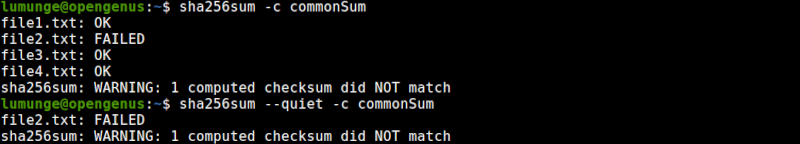Now, we only get errors. This is useful where we only want to display issues.

Using the md5 algorithm we write:

``````\$ md5sum --quiet -c file
``````

Here we should make sure that the checksums we are comparing are generated with the same algorithm, that is MD5.

1. We can also use the --status option to record the exit status in the \$? environment variable. That is, if the checksum matches, our exit status will be 0 otherwise it will be 1 denoting an error:
``````\$ sha256sum --status -c file
``````

And to read the status we write:

``````\$ echo \$?
``````In this example, we initially have an exit status of 1 which is an indication of a failed checksum matching.

This is useful in scripts whereby we evaluate the exit status and proceed accordingly.

After which we correct the file that we previously modified - file2.txt by adding the original content which we used to generate the previous checksums.
When we compare again, we have an exit status of 0 which means we have no errors.

1. The --strict option allows us to exit with a non-zero exit status when we have invalid checksum lines.
Here we write the command as follows.
Using MD5 algorithm:
``````\$ md5sum --strict -c file
``````

Or when using SHA-256 we write:

``````\$ sha256sum --strict -c file
``````
1. Another useful option is the -w option which warns us about checksum lines that are not formatted properly:
``````\$ md5sum -w -c file
``````

In the above example, warning messages are displayed if there are issues with the checksum.

1. To get the md5 checksum of a string in the terminal we write:
``````\$ echo -n [string] | md5sum
``````

And to get the sha256 checksum for a string we write:

``````\$ echo -n [string] | sha256sum
``````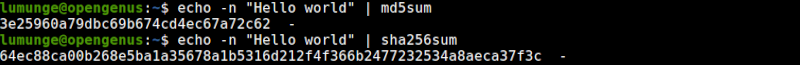This is useful when comparing complex commands, for example, sed, perl, or awk text filtering commands.

1. We can also get the checksum for the file structure of an entire directory. We get the file structure of a directory using the tree command. We pipe the output of this command to the MD5 algorithm or the SHA-256 algorithm to generate a checksum.
Now if the file structure changes, we will be able to know since the checksums won't match.
``````\$ tree | md5sum
``````

or

``````\$ tree | sha256sum
``````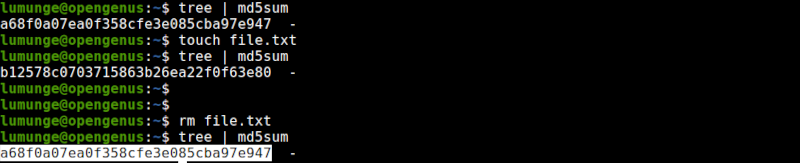## Summary.

MD5 is a cryptographic hashing algorithm we use to create 128-bit strings from an arbitrary string or file.

SHA-256 is a cryptographic hashing algorithm we use to create 256-bit message digests or hashes from arbitrary strings or files.

MD5 although great for checking file integrity, it suffers from collisions. This is whereby we have two different files producing the same hash value, this only happens if there is a practical way to produce collisions on purpose.

We have also stated that two files cannot generate the same hash value except in rare cases where we engineer a collision. Also, SHA256 has far much fewer collisions compared to MD5 where collisions are very rare but possible.

Between the two algorithms MD5 is useful for verifying the integrity of a file or directory while SHA-256 can verify both the integrity and authenticity of a file or directory since it has fewer collisions and is secure.
A drawback of SHA-256 is the fact that it is slow compared to MD5.

For a comprehensive guide on the md5sum or sha256sum commands we can execute the commands \$ man md5sum or \$ man sha256sum to check out their manuals.

#### Erick Lumunge

Erick is a passionate programmer with a computer science background who loves to learn about and use code to impact lives positively.

md5sum and sha256sum commands in Linux